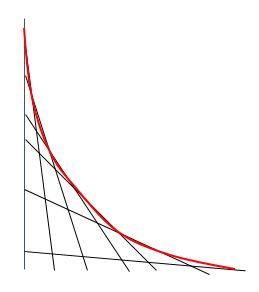# Tangential Network 2

Calculus Level 5Let $n$ be a real number; define the curve $C_n$ by the equation $x^n + y^n = 1.\ \ \ \ x, y \geq 0$ For every point $x, y$ on $C_n$ there is a tangent line. This tangent line intersects both coordinate axes. Consider the line segment between these intersection points.

There exists one curve $C_n$ for which all these tangential line segments have the same length. How much is $n$?

×